## Sunday, 5 October 2014

### EXAMPLES TO DETERMINE THE IMAGE DISTANCE AND MAGNIFICATION

EXAMPLE1 FOR CONCAVE MIRROR
A Star Wars action figure, 8.0 cm tall, is placed 23.0 cm in front of a concave mirror with a focal length of 10.0 cm. Where is the image? How tall is the image? What are the characteristics of the image?
The first step is to draw the ray diagram, which should tell you that the image is real, inverted, smaller than the object, and between the focal point and the center of curvature. The location of the image can be found from the mirror equation: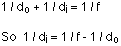which can be rearranged to: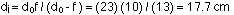The image distance is positive, meaning that it is on the same side of the mirror as the object. This agrees with the ray diagram. Note that we don't need to worry about converting distances to meters; just make sure everything has the same units, and whatever unit goes into the equation is what comes out.
Calculating the magnification gives: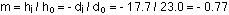Solving for the image height gives:The negative sign for the magnification, and the image height, tells us that the image is inverted compared to the object.
To summarize, the image is real, inverted, 6.2 cm high, and 17.7 cm in front of the mirror.

### The same Star Wars action figure, 8.0 cm tall, is placed 6.0 cm in front of a convex mirror with a focal length of -12.0 cm. Where is the image in this case, and what are the image characteristics?

Again, the first step is to draw a ray diagram. This should tell you that the image is located behind the mirror; that it is an upright, virtual image; that it is a little smaller than the object; and that the image is between the mirror and the focal point.
The second step is to confirm all those observations. The mirror equation, rearranged as in the first example, gives: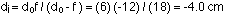Solving for the magnification gives: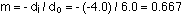This gives an image height of 0.667 x 8 = 5.3 cm.
All of these results are consistent with the conclusions drawn from the ray diagram. The image is 5.3 cm high, virtual, upright compared to the object, and 4.0 cm behind the mirror.